# Chemistry Assignment Help with Kinetics of First Order Reaction

## 4.5 Kinetics of First Order Reaction

A first order reaction is one whose rate varies as 1st power of the concentration of the reactant i.e. the rate increases as number of times as the number of times the concentration of reactant is increased.

Let us consider a uni molecular first order reaction represented by the general equation.

A ___________ Product

A x = 0 … t = 0A – x x = x … t = 0

The initial concentration of A is a mole L–1 and its concentration after any time t is (a – x) mole L–1. This means during the time interval t, x mole L–1 of A has reacted.

The rate of reaction at any time t is given by the following first – order kinetics.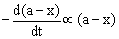or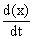µ (a – x)

or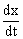= k (a – x)

(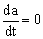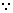a has a given value for a given expt.)

where k is the rate constant of the reaction.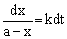This is differential rate equation and can be solved by integration.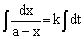or – ln (a – x) = k.t + C …(1)

The constant C can be evaluated by applying the initial condition of the reaction i.e. when t = 0, x = 0. Putting these in equation 1, we get

C = – lna

Putting the value of C in equation 1, we get

ln (a – x) = k.t. – lna or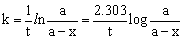…(2)

If [A0] and [A] be the concentrations of reactant at zero time and time t, respectively then Eq. 2 may be put as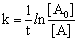The rate constant units for nth order reaction is as follows.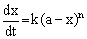or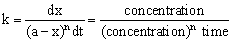= conc. 1–n . time–1

4.5.1 Half-time or half-life period of a first order reaction

The half-time of a reaction is defined as the time required to reduce the concentration of the reactant to half of its initial value. It is denoted by the symbol t1/2. Thus,

When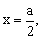t = t1/2

Putting these in equation 2 mentioned above, we get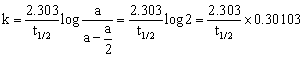(log 2 = 0.30103)

t1/2 =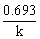…(3)

Since k is a constant for a given reaction at a given temperature and the expression lacks any concentration term so from equation 3 it is evident that half-time of a 1st order reaction is a constant independent of initial concentration of reactant.### Email Based Assignment Help in Kinetics of First Order Reaction

We are the leading online Assignment Help provider. Find answers to all of your doubts regarding the Chemical Kinetics in chemistry. We at assignmenthelp.net provide homework, Assignment Help to the school, college or university level students. Our expert online tutors are available to help you in Chemical Kinetics. Our service is focused on: time delivery, superior quality, creativity, originality and plagiarism free.